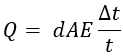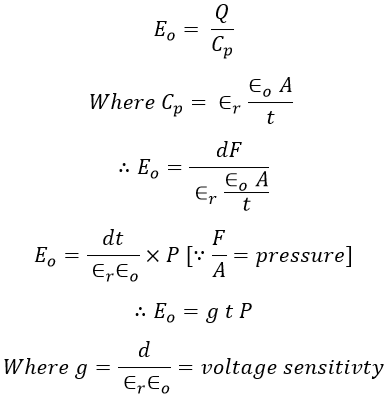# Piezoelectric Transducer - Working Principle, Theory & Applications

## What is Piezoelectric Transducer?

The piezoelectric transducer is an active transducer that converts physical quantity (force or pressure or stress) into an electric potential. The piezoelectric transducer consists of a piezoelectric crystal made up of piezoelectric material which develops electrical potential across its surface on application mechanical stress. They are self-generating transducers.

The electrical energy generated is proportional to the applied mechanical force. On the application of mechanical force on the surface of the piezoelectric crystal. A charge will be produced on the surface of the crystal, this results in creating a potential difference between the two surfaces of the crystal thus inducing an electric potential.

Since the voltage produced is the function of applied force or pressure or mechanical stress. By connecting a voltage measuring instrument across the surface of the crystal. The amount of mechanical stress experienced by the crystal can be determined.

In this way, a physical quantity can be measured with the help of a piezoelectric transducer. In this process of conversion, there is no requirement of an external source of supply, thus the transducer is said to be called an active transducer.

## Working Principle of Piezoelectric Transducer :

The piezoelectric transducer work on the principle of the piezoelectric effect. The materials used for the construction of piezoelectric crystal is quartz, rochelle salt, dipotassium tartrate, potassium dihydrogen phosphate, ammonium dihydrogen phosphate. etc, these materials are called piezoelectric materials.

When a piezoelectric crystal is subjected to force or mechanical stress an electric potential is developed on the surface of the crystal. This is due to the displacement of charges in the crystal.

Conversely, if an electrical voltage is given, there will be deformation in the shape of the crystal by changing its dimensions. This process of conversion of force or pressure or mechanical stress into electrical energy or vice versa is refer as the piezoelectric effect.

Let, F be the force applied on the surface of the crystal. The magnitude and polarity of the developed surface potential are proportional to the magnitude and direction of F. Here the developed potential (charge) polarity depends on the direction of F.

#### The charge developed by the crystal is given by,Q = d × F (in coulombs) ...(1)Where,F = Force applied in Newtonsd = Charge sensitivity of the crystal.For a given crystal the charge sensitivity d is constant. The thickness of the piezoelectric crystal changes due to the applied force. Therefore,Where,A = Area of the piezoelectric crystal in m2E = Young's modulus in N/m3t = Thickness of the piezoelectric crystal in m.The young's modulus E can be defined as the ratio of stress to strain i.e.,Where,A = area = lbl = Length of the crystal in mb = Width of the crystal in mBy substituting equation 2 in 1 we get,Due to this charge at the electrode, an output voltage Eo will be generated which can be given by,For a given crystal the voltage sensitivity is constant and is expressed in units of Vm/N. Now, from the equation of output voltage Eo,Now, P is the applied pressure or stress to the crystal and ∈ is the electric field intensity in the crystal. Therefore, the voltage sensitivity of the crystal can be stated as the ratio of electric field intensity to applied pressure or stress.

Also, the direction of the polarity of the induced emf depends upon the direction of applied force or pressure. Hence, the magnitude and direction of the induced potential depend upon the direction and magnitude of the applied force or pressure.

## Modes of Operation of Piezoelectric Crystal :

The piezoelectric crystal can be operated in different modes such as thickness-shear mode, thickness expansion mode, transverse expansion mode, face shear mode, etc as shown below.

When two crystals are bonded together such that, the electrical axes of the crystals are perpendicular to each other, the resulting structure is called 'twisters' or 'benders'. When a twister is subjected to a twisting motion a voltage will be generated at the output.

Similarly, when a bender is subjected to a bending motion, a voltage will be generated at the output. The curvatures of twister and bender piezoelectric transducers when subjected to voltage are shown in the below figure.

Apart from these a piezoelectric crystal also be used in another mode of operation to carry out the measurement of force. In order to measure the force, a thin quartz plate is employed in a crystal-controlled electronic oscillator. In this method, the frequency of electrical oscillations is known by the natural frequency of mechanical oscillations of the quartz plate.

## Equivalent Circuit of Piezoelectric Transducer :

The below figure shows the equivalent circuit of a piezoelectric transducer.

Let a piezoelectric crystal is used as a piezoelectric transducer and the transducer employs a charge generator as its source as shown in figure (i). Therefore, the charge developed by the crystal is given by,

Q = d × F

Figure (ii) contains a voltage source that is equivalent to the charge generator i.e., fig (i) is equivalent to fig (ii). Therefore voltage developed by the voltage source is given by,

Vo = Q/Cp = dF/Cp

## Applications of Piezoelectric Transducers :

#### The desirable features of piezoelectric materials are stability, insensitivity to changes in humidity, temperature, high output. When compared to all other materials quartz has the highest stability (but provides low output). Therefore, it is widely used in stabilizing electronic oscillators.The piezoelectric materials and transducers are used mainly for dynamic measurements and cannot be used for static pressure of strain measurements.There can be used in the process which requires measurement of high pressure.Piezoelectric materials can be applied in those systems which require measured variables in electrical form.Barium titanate, one of the piezoelectric materials employed in ultrasonic generators. Used in industrial cleaning equipment, and in sonar (sonar is an underwater detection system).Piezoelectric materials are used in accelerometers.Piezoelectric elements can be used in vibration pick-up.

Do not enter any spam links and messages